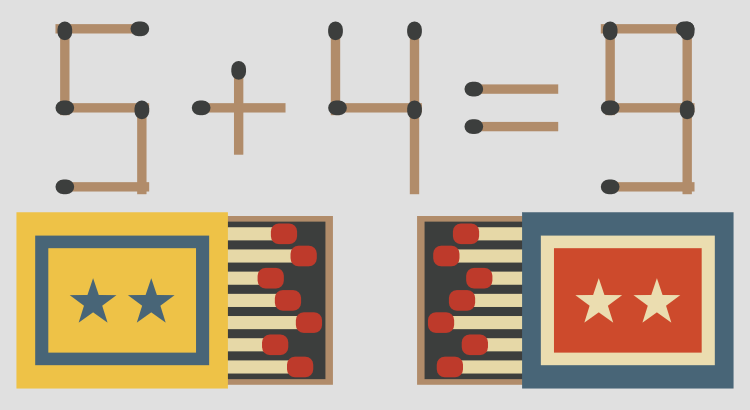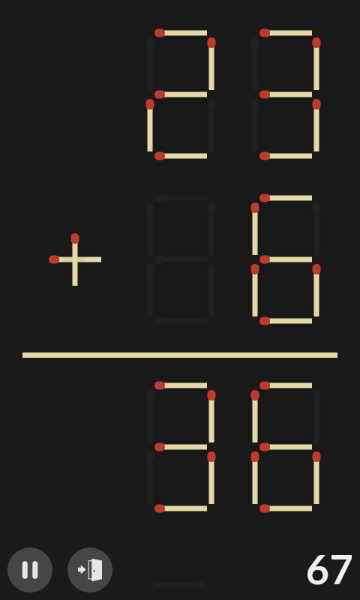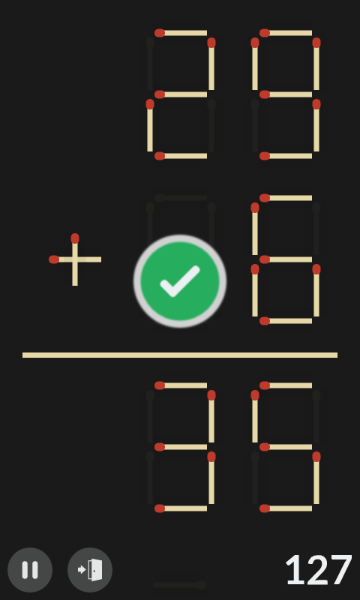# Matchsticks: Endless Digit Hunter

Matchsticks: Endless Digit Hunter is an HTML5 one-button matchstick (aka toothpick) puzzle/logic game in which your goal is to rearrange all given math equations and make them true by moving just one match.

## Instructions

The game can be played on 4 different levels of difficulty:

1. Normal – the equations contain numbers from 0 to 9.
2. Medium – the equations contain numbers from 0 to 19.
3. Hard – the equations contain numbers from 0 to 49.
4. Insane – the equations contain numbers from 0 to 99.

On each level there are 5 faulty math equations which must be fixed as fast as possible!

All equations are procedurally generated so the levels are always different.
To make the given equation true, tap on a match and move it to the right place.

For each bad move you get a penatly of 10 seconds!